## KNN算法解析_墨小灯的博客-程序员宅基地

KNN算法网上的给出的解释很多，源码也很多，有许多写的很好，所以这个仅作个人理解之用。

KNN算法简介：

KNN算法采用测量不同特征值之间的距离方法进行分类。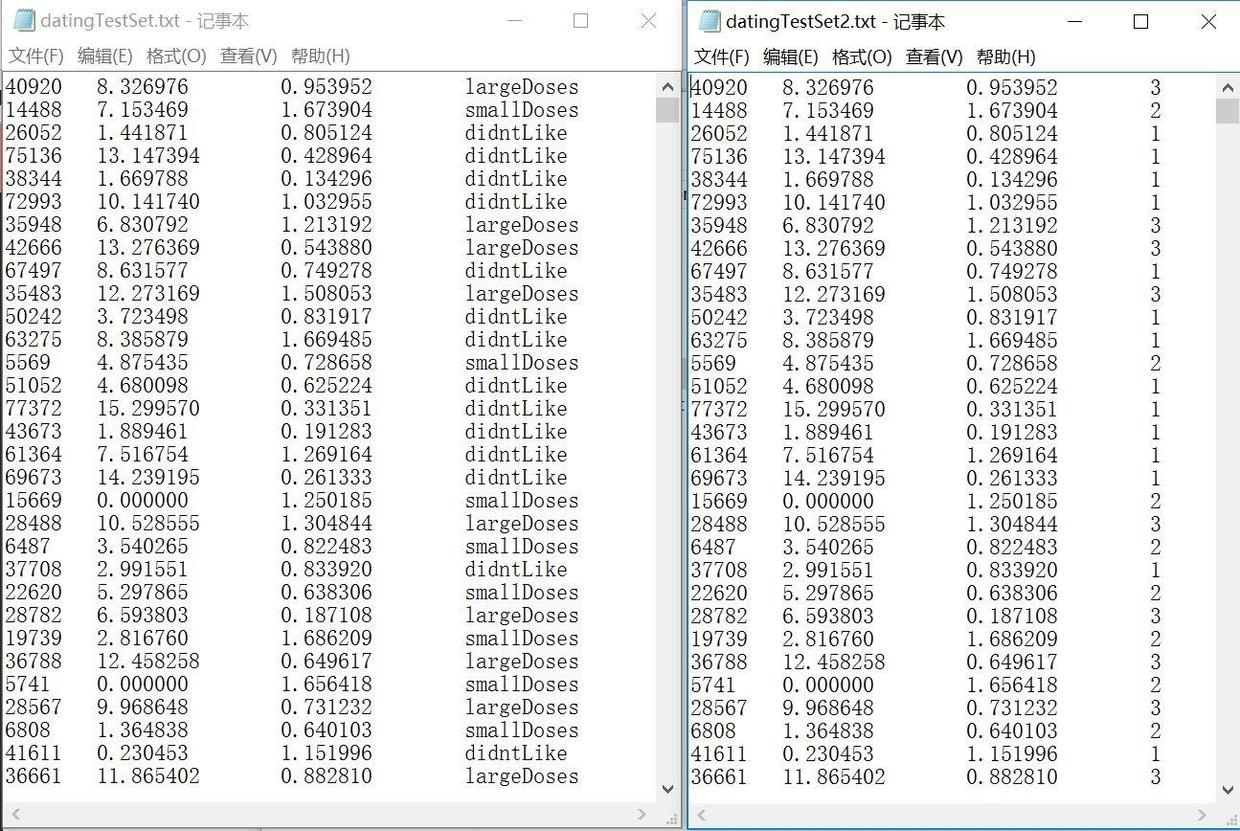``````#将评价转化为数字

if listFromLine == 'largeDoses':
listFromLine =3
elif listFromLine == 'smallDoses':
listFromLine=2
else:
listFromLine=1
``````

``````# -*- coding: utf-8 -*-
from numpy import *
import matplotlib.pyplot as plt

def file2matrix(filename):
fr = open(filename,'r')
numberOfLines = len(arrayOlines)
returnMat = zeros((numberOfLines,3)) #构造全零阵来存放数
classLabelVector = [] #开辟容器
index = 0

for line in arrayOlines:
#清洗数据
line = line.strip()
listFromLine = line.split('\t')
#将评价转化为数字
if listFromLine == 'largeDoses':
listFromLine =3
elif listFromLine == 'smallDoses':
listFromLine=2
else:
listFromLine=1
#存入数据到list
returnMat[index,:] = listFromLine[0:3] #三个特征分别存入一行的三个列
classLabelVector.append(int(listFromLine)) #最后一行是类别标签

index +=1
return returnMat,classLabelVector

#将喜欢强度转化为颜色
def ColorOfDatingLable(num):
datingLabels_rgb = []
for i in range(len(num)):
if num[i]==3:
datingLabels_rgb.append('red')
elif num[i]==2:
datingLabels_rgb.append('green')
else:
datingLabels_rgb.append('black')

return datingLabels_rgb

datingDataMat,datingLabels = file2matrix('D:\\PYthon-learning\\Python_test\\knn-test-2\\KNN\\datingTestSet.txt')

##################创建图表1#####################
plt.figure(1) #创建图表1

ax1 = plt.subplot(1,2,1) # 图表1中创建子图1
plt.title("original color")
plt.xlabel('play game/time %')
plt.ylabel('ice cream cost/week')

ax2 = plt.subplot(1,2,2) # 图表1中创建子图2
plt.title("improved color")
plt.xlabel('play game/time %')
plt.ylabel('ice cream cost/week')

###################创建图表2####################
plt.figure(2) #创建图表2

ax3 = plt.subplot(2,2,1) # 图表2中创建子图1
plt.title("play game & ice cream cost")
plt.xlabel('play game/time %')
plt.ylabel('ice cream cost/week')

ax4 = plt.subplot(2,2,2) # 图表2中创建子图2
plt.title("fly distance & play game")
plt.xlabel('fly distance/year')
plt.ylabel('play game/time %')

ax5 = plt.subplot(2,2,3) # 图表2中创建子图2
plt.title("fly distance & ice cream cost")
plt.xlabel('fly distance/year')
plt.ylabel('ice cream cost/week')

#plt.scatter(x[i],y[i],marker = 样式,s=大小半径,color =(np.random.rand(1,3)),label = str(i+1))
ax1.scatter(datingDataMat[:,1],datingDataMat[:,2],15*array(datingLabels),15*array(datingLabels)) #scatter散点图展示第二列和第三列数据
ax2.scatter(datingDataMat[:,1],datingDataMat[:,2],s=15*array(datingLabels),color=ColorOfDatingLable(datingLabels)) #scatter散点图展示第二列和第三列数据,第一个15*array(datingLabels)用来表现不同标签的不同半径
ax3.scatter(datingDataMat[:,1],datingDataMat[:,2],s=15*array(datingLabels),color=ColorOfDatingLable(datingLabels),label='largeDoses/smallDoses/didntLike')
ax4.scatter(datingDataMat[:,0],datingDataMat[:,1],s=15*array(datingLabels),color=ColorOfDatingLable(datingLabels),label='largeDoses/smallDoses/didntLike')
ax5.scatter(datingDataMat[:,0],datingDataMat[:,2],s=15*array(datingLabels),color=ColorOfDatingLable(datingLabels),label='largeDoses/smallDoses/didntLike')

ax3.legend(loc='upper right')
ax4.legend(loc='upper right')
ax5.legend(loc='upper right')

plt.show()

``````

``````def file2matixr（filename）#用于读取文本文件中的数据 ；

fr = open(filename,'r')#打开文件filename;

# 计算文本文件的行数

returnMat = zeros((numberOfLines,3)) #构造全零阵来存放数

classLabelVector = [] # 创建类标签  /开辟容器

index = 0  # 定义索引

for line in arrayOlines:# 读取文件的每一行并处理

#清洗数据

line = line.strip()  # 去除行的尾部的换行符

listFromLine = line.split('\t')  # 将一行数据按空进行分割

if listFromLine == 'largeDoses':
listFromLine =3
elif listFromLine == 'smallDoses':
listFromLine=2
else:
listFromLine=1 #将评价转化为数字

#然后，存入数据到list

returnMat[index,:] = listFromLine[0:3] #三个特征分别存入一行的三个列
classLabelVector.append(int(listFromLine)) #最后一行是类别标签

#至此，循环结束

return returnMat,classLabelVector# 返回数据集和对应的类标签
``````
``````#将喜欢强度转化为颜色
def ColorOfDatingLable(num):
datingLabels_rgb = []
for i in range(len(num)):
if num[i]==3:
datingLabels_rgb.append('red')
elif num[i]==2:
datingLabels_rgb.append('green')
else:
datingLabels_rgb.append('black')

return datingLabels_rgb

datingDataMat,datingLabels = file2matrix('D:\\PYthon-learning\\Python_test\\knn-test-2\\KNN\\datingTestSet.txt')``````

``````plt.figure(1) #创建图表1

ax1 = plt.subplot(1,2,1) # 图表1中创建子图1
plt.title("original color")
plt.xlabel('play game/time %')
plt.ylabel('ice cream cost/week')

ax2 = plt.subplot(1,2,2) # 图表1中创建子图2
plt.title("improved color")
plt.xlabel('play game/time %')
plt.ylabel('ice cream cost/week')

###################创建图表2####################
plt.figure(2) #创建图表2

ax3 = plt.subplot(2,2,1) # 图表2中创建子图1
plt.title("play game & ice cream cost")
plt.xlabel('play game/time %')
plt.ylabel('ice cream cost/week')

ax4 = plt.subplot(2,2,2) # 图表2中创建子图2
plt.title("fly distance & play game")
plt.xlabel('fly distance/year')
plt.ylabel('play game/time %')

ax5 = plt.subplot(2,2,3) # 图表2中创建子图2
plt.title("fly distance & ice cream cost")
plt.xlabel('fly distance/year')
plt.ylabel('ice cream cost/week')``````

pyplot是python绘图的一个工具，pyplot是一个有状态的对象，包含了当前的图，画图区域，等。

p1 = plt.subplot(211) 或者 p1 = plt.subplot(2,1,1)， 表示创建一个2行，1列的图，p1为第一个子图.

``````#plt.scatter(x[i],y[i],marker = 样式,s=大小半径,color =
(np.random.rand(1,3)),label = str(i+1))

ax1.scatter(datingDataMat[:,1],datingDataMat[:,2],15*array(datingLabels),15*array(datingLabels))

#scatter散点图展示第二列和第三列数据

ax2.scatter(datingDataMat[:,1],datingDataMat[:,2],s=15*array(datingLabels),color=ColorOfDatingLable(datingLabels))

#scatter散点图展示第二列和第三列数据,第一个15*array(datingLabels)用来表现不同标签的不同半径

ax3.scatter(datingDataMat[:,1],datingDataMat[:,2],s=15*array(datingLabels),color=ColorOfDatingLable(datingLabels),label='largeDoses/smallDoses/didntLike')

ax4.scatter(datingDataMat[:,0],datingDataMat[:,1],s=15*array(datingLabels),color=ColorOfDatingLable(datingLabels),label='largeDoses/smallDoses/didntLike')

ax5.scatter(datingDataMat[:,0],datingDataMat[:,2],s=15*array(datingLabels),color=ColorOfDatingLable(datingLabels),label='largeDoses/smallDoses/didntLike')

ax3.legend(loc='upper right')
ax4.legend(loc='upper right')
ax5.legend(loc='upper right')

plt.show()``````

``````#导入必要的模块

import numpy as np
import matplotlib.pyplot as plt

#产生测试数据
x = np.arange(1,10)
y = x

#产生figure对象

fig = plt.figure()

#设置标题
ax1.set_title('Scatter Plot')

#设置X轴标签
plt.xlabel('X')

#设置Y轴标签
plt.ylabel('Y')

#画散点图
ax1.scatter(x,y,c = 'r',marker = 'o')

#设置图标
plt.legend('x1')

#显示所画的图
plt.show()
``````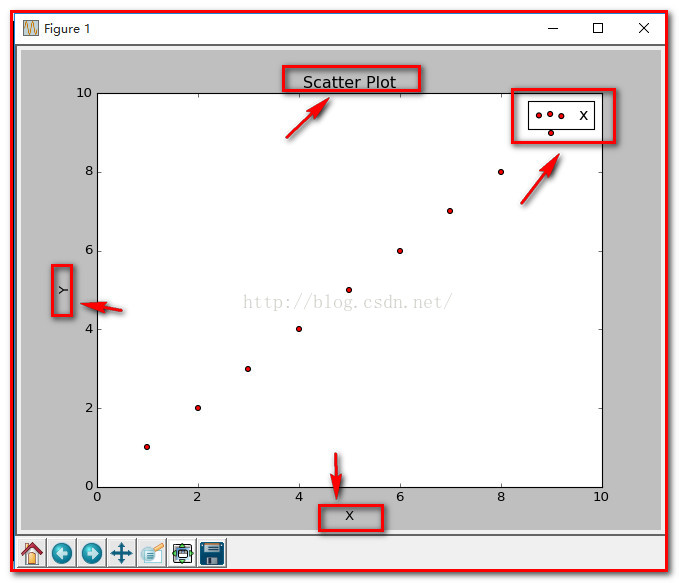shape函数是numpy.core.fromnumeric中的函数，它的功能是查看矩阵或者数组的维数。

``````>>> e = eye(3)
>>> e
array([[ 1.,  0.,  0.],
[ 0.,  1.,  0.],
[ 0.,  0.,  1.]])
>>> e.shape
(3, 3)  ``````

figure1：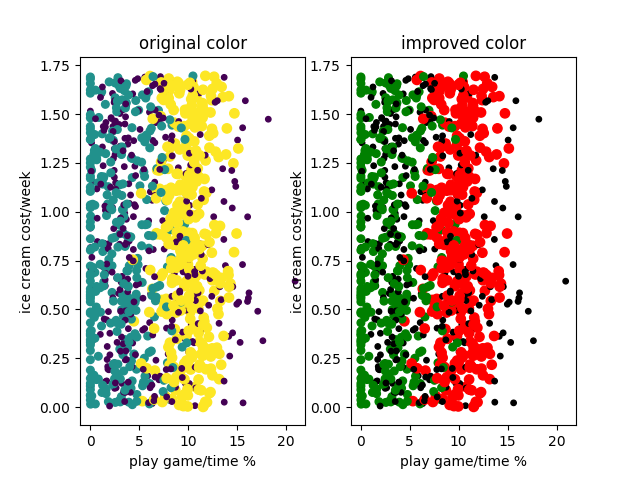``````#plt.scatter(x[i],y[i],marker = 样式,s=大小半径,color =颜色,label = 点备注

#15*array(datingLabels)它来规定点的尺度大小
``````

figure 2：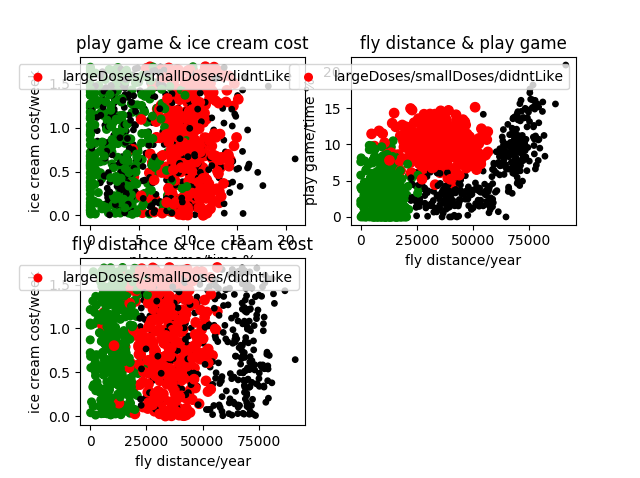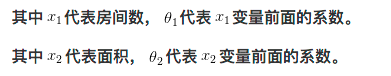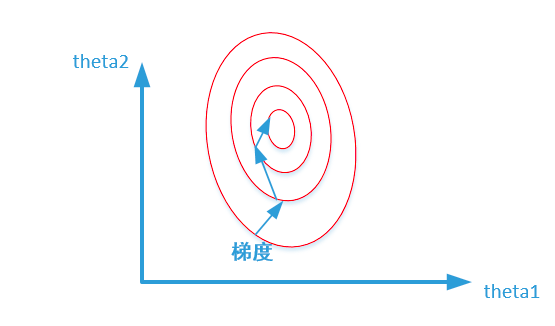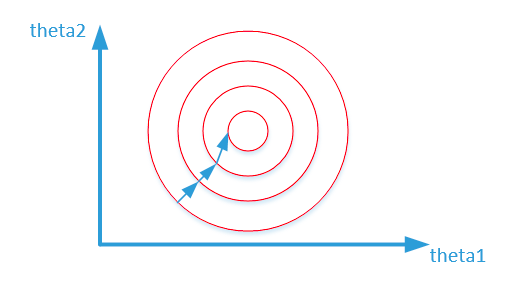``````# 归一化函数
def autoNorm(dataSet):

# 求数据矩阵每一列的最小值
minVals = dataSet.min(0)

# 求数据矩阵每一列的最大值
maxVals = dataSet.max(0)

# 求数据矩阵每一列的最大最小值差值
ranges = maxVals - minVals

# 返回数据矩阵第一维的数目
m = dataSet.shape

# 求矩阵每一列减去该列最小值，得出差值
normDataSet = dataSet - tile(minVals,(m,1))

# 用求的差值除以最大最小值差值，即数据的变化范围，即归一化
normDataSet = normDataSet / tile(ranges,(m,1))

# 返回归一化后的数据，最大最小值差值，最小值  ``````

1.把数据集分类测试集和训练集，当然，knn没有训练这个说法
2.测试集遮去标签，只输入数据，直接靠KNN的算法，进行预测判断标签
3.测试集本身自己的标签是正确的，只是暂时不用而已，用来当判断knn算法是否判断正确
4.错误率也就是=贴错的标签总数/总的测试样本数

``````def classify0(inX,dataSet,labels,k): # inX用于需要分类的数据，dataSet输入训练集

######输入与训练样本之间的距离计算######
dataSetSize = dataSet.shape # 读取行数,shape则为列数
diffMat = tile(inX,(dataSetSize,1))-dataSet # tile,重复inX数组的行(dataSize)次，列重复1
sqDiffMat = diffMat**2 #平方操作
sqDistances = sqDiffMat.sum(axis=1) # 每一个列向量相加,axis=0为行相加
distances = sqDistances**0.5

sortedDistIndicies = distances.argsort() # argsort函数返回的是数组值从小到大的索引值
#print sortedDistIndicies #产生的是一个排序号组成的矩阵
classCount={}

######累计次数构成字典######
for i in range(k):
voteIlabel = labels[sortedDistIndicies[i]] #排名前k个贴标签
classCount[voteIlabel] = classCount.get(voteIlabel,0)+1 # 不断累加计数的过程，体现在字典的更新中

#get(key,default=None),就是造字典

######找到出现次数最大的点######
sortedClassCount = sorted(classCount.iteritems(),key = operator.itemgetter(1),reverse=True)
#以value值大小进行排序，reverse=True降序
#key = operator.itemgetter(1)，operator.itemgetter函数获取的不是值，而是定义了一个函数，通过该函数作用到对象上才能获取值

return sortedClassCount
#返回出现次数最多的value的key

``````

``````#测试分类器精度
def datingTest(HORATIO,K):
hoRatio = HORATIO #取百分之十作为测试数据
datingDataMat,datingLabels = file2matrix("D:\PYthon-learning\Python_test\knn-test-2\KNN\datingTestSet2.txt")
normMat,ranges,minVals = autoNorm(datingDataMat)
m = normMat.shape
numTestVecs = int(m*hoRatio) #挑选出多少组测试数据
errorCount = 0.0

for i in range(numTestVecs):
classifierResult = classify0(normMat[i,:],normMat[numTestVecs:m,:],datingLabels[numTestVecs:m],K)

print "the classifier came back with:%d,the real answer is %d"%(classifierResult,datingLabels[i])
if classifierResult !=datingLabels[i]:
errorCount +=1.0
print "the total error rate is : %f" % (errorCount/float(numTestVecs))

if __name__ == '__main__':
HORATIO = input("Please enter test set (%all): ")
K = input("Please enter the k: ")
datingTest(HORATIO,K)
``````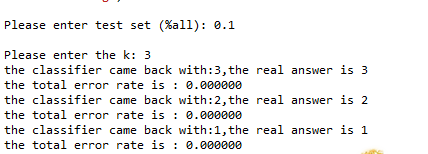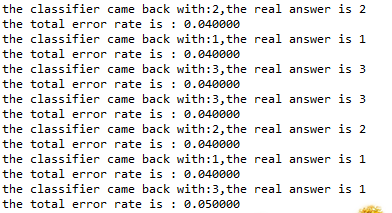``````def classifyPerson():
resultList = ['not at all','in small doses','in large doses']
percentTats = input("percentage of time spent playing video games?")
ffMiles = input("frequent flier miles earned per year?")
iceCream = input("liters of ice cream consumed per year?")
datingDataMat,datingLabels = file2matrix("D:\PYthon-learning\Python_test\knn-test-2\KNN\datingTestSet2.txt")
normMat,ranges,minVals = autoNorm(datingDataMat)
inArr =array([ffMiles,percentTats,iceCream])
classifierResult = classify0((inArr-minVals)/ranges,normMat,datingLabels,3)
print "You will probably like this person: ",resultList[classifierResult -1]

if __name__ == '__main__':
classifyPerson()

``````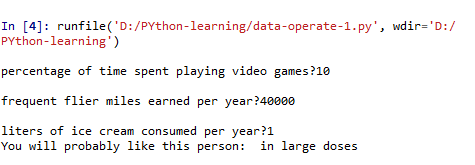### Android 应用快捷方式 Shortcuts(一)_tracydragonlxy的博客-程序员宅基地

Android 应用快捷方式 Shortcuts简介： 快捷方式可帮助用户快速访问您的应用的某些部分，从而为他们呈现特定类型的内容。一次最多可以为应用发布五个快捷方式（静态和动态快捷方式加在一起），但大多数启动器只能显示四个。不过，用户可创建的应用固定快捷方式数量没有限制。应用无法移除固定快捷方式，但仍然可以停用它们。效果图：快捷方式的类型：静态快捷方式：最适合在用户与应用互动的整个生命周期内使用一致结构链接到内容的应用。 由于大多数启动器一次只能显示四个快捷方式，因此静态快捷方式对常见 A

### word 2010中设置默认粘贴为 只保留文本粘贴【visio也适用于快捷键方式】_weixin_30722589的博客-程序员宅基地

VISIO也适用如下方式：3. 当然也可以直接是 Ctrl + Alt + V打开选择性粘贴选项卡 来选择其中某项来粘贴也是可以的转：word 2010中设置默认粘贴为 只保留文本粘贴2012年12月21日 21:19:32 小龙王2010 阅读数 6786更多分类专栏： Microsoft Office ...

### mysql8 slap_【原创】mysqlslap 使用总结_weixin_39646628的博客-程序员宅基地

mysqlslap 可以用于模拟服务器的负载，并输出计时信息。其被包含在 MySQL 5.1 的发行包中。测试时，可以指定并发连接数，可以指定 SQL 语句。如果没有指定 SQL 语句，mysqlslap 会自动生成查询 schema 的 SELECT 语句。1. 查看帮助信息。[[email protected] libmysql]# mysqlslap --helpmysqlslap Ver 1.0 Di...

### Android学习笔记(48):Android应用的资源_这波lucio来全学了的博客-程序员宅基地

Android应用资源的两大类：assets目录下，无法通过R资源清单类访问的原生资源，应用程序需要通过AssetManager以二进制流的形式读取资源。res目录下，可通过R资源清单访问的资源，Android SDK会在编译应用时，自动在R.java文件中为这些资源建立索引。

### 性能工具列表_weixin_30642561的博客-程序员宅基地

Load and Performance Test ToolsBrowserMob- On-demand, self-service, low-cost, pay-as-you-go service enables simulation of large volumes of real browsers hitting a website. Utilizes Amazon Web Servi...

### linux RDP 共享磁盘,USB Over Network - USB Server for Linux (RDP使用讀卡機殘念)_永远的12的博客-程序员宅基地

USB Over NetworkLinux上常遇到讀卡機無法在 !FireFox 使用的問題，其實這個議題已經有許多的商用軟體已經解決了。許多軟體提供透過驅動程式與網路將裝置掛載到遠端的作業系統中，從下面的網址可以看到現有的 USB Over Network Softwarehttp://usb-over-network.qarchive.org/USB Server如何將本地端的 USB 連接埠...